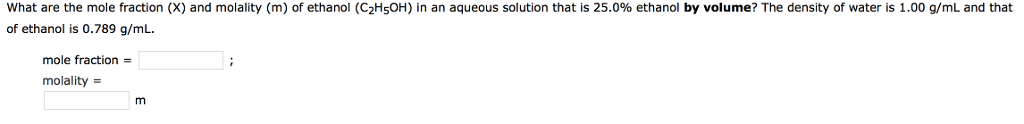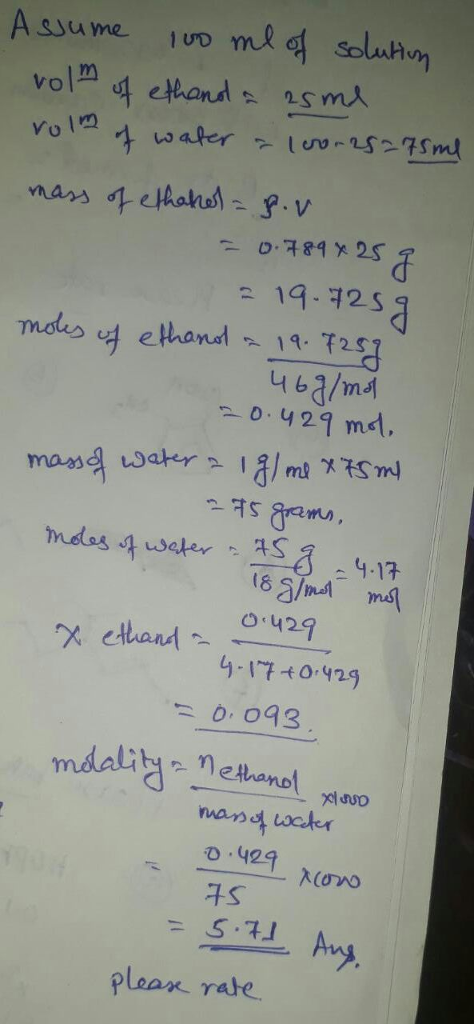# Question & Answer: What are the mole fraction (X) and molality (m) of ethanol (C_2H_5OH) in an aqueous…..What are the mole fraction (X) and molality (m) of ethanol (C_2H_5OH) in an aqueous solution that is 25.0% ethanol by volume? The density of water is 1.00 g/mL and that of ethanol is 0.789 g/mL. mole fraction =: molality = m